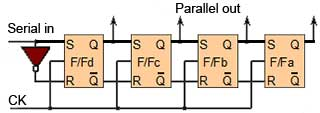# Sequential Logic## Introduction

The logic circuits discussed in Digital Electronics Module 4 had output states that depended on the particular combination of logic states at the input connections to the circuit. For this reason these circuits are called combinational logic circuits.

Use Module 5 to learn about digital circuits that use SEQUENTIAL LOGIC.

In these circuits the output depends, not only on the combination of logic states at its inputs, but also on the logic states that existed previously. In other words the output depends on a SEQUENCE of events occurring at the circuit inputs. Examples of such circuits include clocks, flip-flops, bi-stables, counters, memories, and registers. The actions of these circuits depend on a range of basic sub-circuits.

## Clock CircuitsModule 5.1 deals with clock oscillators, which are basically types of square wave generators or oscillators that produce a continuous stream of square waves or a continuous train of pulses (a "square" wave whose mark to space ratio is NOT 1:1). These pulses are used to sequence the actions of other devices in the sequential logic circuit so that all the actions taking place in the circuit are properly synchronised.

## Bi-Stable Logic DevicesBi-stable devices (popularly called Flip-flops) described in Modules 5.2 to 5.4, are sub-circuits, usually contained within ICs, and are the most basic type of 1-bit memory. They have outputs that can take up one of two stable states, Logic 1 or logic 0 or off. Once the device is triggered into one of these two states by an external input pulse, the output remains in that state until another pulse is used to reverse that state, so that a logic 1 output becomes logic 0 or vice versa. Again the circuit remains stable in this state until an input signal is used to reverse the output state. Hence the circuit is said to have Bi (two) stable output states.## Counters

Various types of digital counters are described in Module 5.6. Consisting of arrangements of bi-stables, they are very widely used in many types of digital systems from computer arithmetic to TV screens, as well as many digital timing and measurement devices.## Registers

Also consisting of arrays of bi-stable elements, the shift registers described in Module 5.7 are temporary storage devices (memories) for multi-bit digital data. The data can be stored in the register either one bit at a time (serial input) or as one or more bytes at a time (parallel input).

The register can then output the data in either serial or parallel form. Shift registers are vital to receiving or transmitting data in digital communications systems. They can also be used in digital arithmetic for operations such as multiplication and division.## A Simple ALU

A simple arithmetic and logic unit (ALU) is described in Module 5.8 and combines many of the combinational and sequential logic circuits described in modules 4 and 5 to demonstrate how a very complex application is built by combining a number of much simpler digital sub circuits.

Top of Page